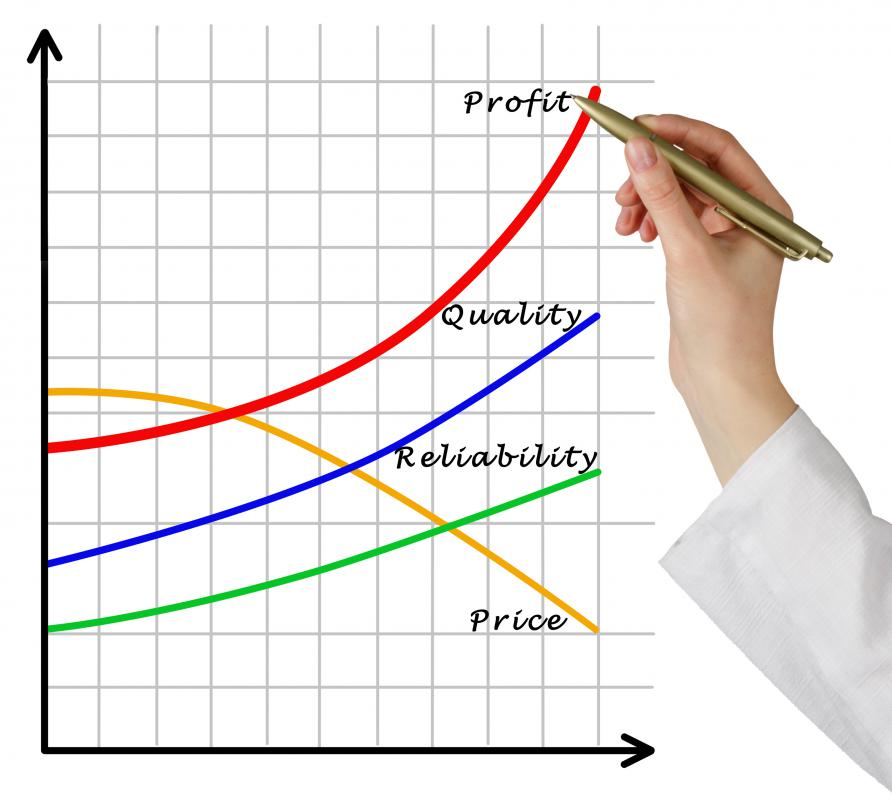# What is the Relationship Between Gross Margin and Profit Margin?

Osmand Vitez

Gross margin and profit margin are two measurements used to determine the strength of a company’s earning power. Gross margin measures how much income a company generates after deducting the cost of goods sold. This is a top-level measurement for a company’s sales figures. The profit margin measures the net income against total sales for a period. Profit margin represents how much money from total sales a company can reinvest into its operations.Gross margin and profit margin are calculated to ascertain a company’s earning power.

A basic formula for gross margin is revenue less cost of goods sold divided by revenue. This returns a percentage that indicates how much revenue goes to pay for the cost of goods sold. For example, a company has \$750,000 US Dollars (USD) in sales and \$450,000 USD in cost of goods sold. This results in a 40 percent gross margin; therefore, \$.60 USD of every dollar goes to pay for the cost of goods sold. The relationship between gross margin and profit margin begins with this calculation.

The profit margin formula is net income divided by total revenue. For example, the company in the previous example has net income of \$75,000 USD with total revenue of \$750,000 USD. The profit margin is 10 percent. The measurement dictates that, for every \$1 USD in sales, \$.10 USD will result in profit for the firm. Companies often compare their gross margin and profit margin to determine the drop in gross profit after expenses.

Both the information for the gross margin and profit margin formulas comes from information on a company’s income statement. Companies prepare this statement each month. This allows for a comparison between these two formulas on a frequent basis. Owners and managers can compare these formulas to determine a trend of increasing or decreasing profitability. Companies can also use the information from these formulas as a benchmark comparison against other firms or the industry standard.

Both gross margin and profit margin can result in flawed figures, sometimes stemming from improperly prepared income statements. The income statement also includes non-cash expenses, like depreciation and amortization. This reduces the profit for a company during a particular period. The profit margin then reflects a lower percentage, providing an inaccurate figure.

External stakeholders are often most interested in these figures. They can calculate the information easily from released financial information. Using this data, investors can determine if they desire to remain invested in the firm or move on to more profitable investments.

## You might also Like